## Calculate the molecular weight of a dibasic acid.0.56gm of which is required 250ml of N/20 sodium hydroxide solution for neutralization.​

Question

Calculate the molecular weight of a dibasic acid.0.56gm of which is required 250ml of N/20 sodium hydroxide solution for neutralization.​

in progress 0
6 months 2021-07-13T19:43:48+00:00 1 Answers 5 views 0

1. Answer: The molecular weight of the dibasic acid is 89.6 g/mol

Explanation:

Normality is defined as the amount of solute expressed in the number of gram equivalents present per liter of solution. The units of normality are eq/L. The formula used to calculate normality: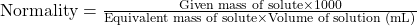….(1)

We are given:

Normality of solution =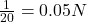Given mass of solute = 0.56 g

Volume of solution = 250 mL

Putting values in equation 1, we get: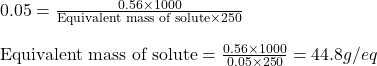Equivalent weight of an acid is calculated by using the equation: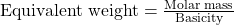…..(2)

Equivalent weight of acid = 44.8 g/eq

Basicity of an acid = 2 eq/mol

Putting values in equation 2, we get: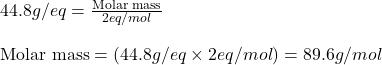Hence, the molecular weight of the dibasic acid is 89.6 g/mol